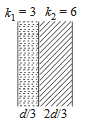#### A parallel plate capacitor with air between the plates has a capacitance of  9pF. The separation between its plates is d . The space between the plates is now filled with two dielectrics. One of the dielectrics has dielectric constan  k1 = 3  and thickness  d/3 while the other one has dielectric constant k2 = 6 and thickness 2d/3   Capacitance of the capacitor is now Option 1)Option 2)Option 3)Option 4)As we learnt in

Parallel Plate Capacitor -- wherein

Area - A seperation between two plates.

If K filled between the plates -- whereinWith dielectric,

(as they are in series)

Option 1)Incorrect

Option 2)Incorrect

Option 3)Incorrect

Option 4)Correct

#### divya.saini